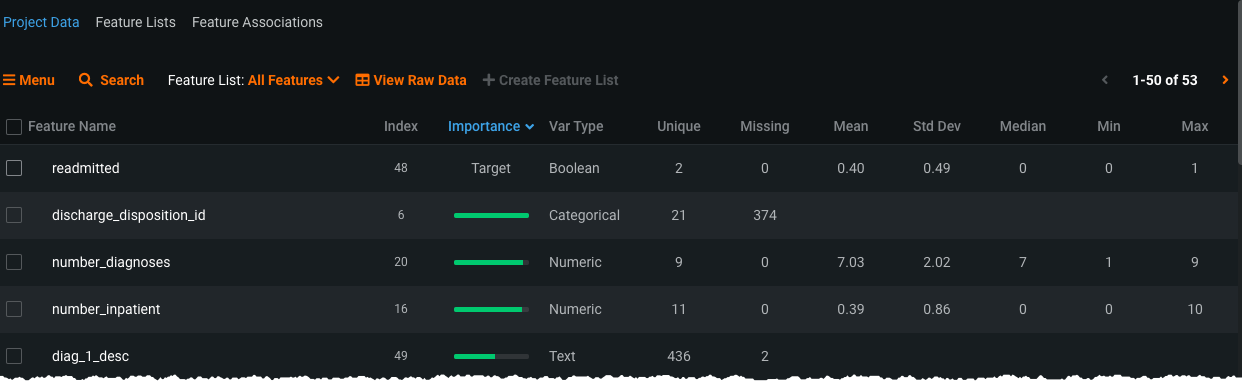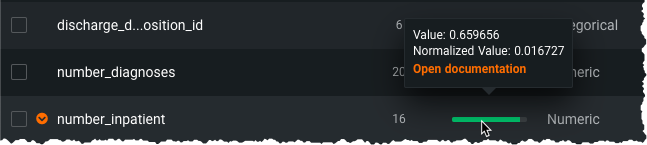Click in-app to access the full platform documentation for your version of DataRobot.

# Modeling process¶

This section provides more detail to help understand DataRobot's initial model building process.

DataRobot also runs a complete data quality assessment that automatically detects, and in some cases addresses, data quality issues. See also the basic modeling process section for a workflow overview.

## Modeling modes¶

The exact action and options for a modeling mode are dependent on your data. In addition to the basic description, the following sections describe circumstantial modeling behavior.

### Small datasets¶

Autopilot for AutoML changes the sample percentages run depending on the number of rows in the dataset. The following table describes the criteria:

Number of rows Percentages run
Less than 2000 Final Autopilot stage only (64%)
Between 2001 and 3999 Final two Autopilot stages (32% and 64%)
4000 and larger All stages of Autopilot (16%, 32%, and 64%)

### Quick Autopilot¶

Quick Autopilot is the default modeling mode. Quick has been optimized to ensure, typically, availability of more accurate models without sacrificing variety of tested options. As a result, reference models are not run.

When used, DataRobot runs the following models during the first stage of Quick (32%) on a "typical" dataset (other cases are described below):

• TensorFlow
• XGBoost
• LightGBM
• Elastic Net, Ridge regressor, or Lasso regressor (text-capable)
• Nystroem Kernel SVM
• Random Forest
• Vowpal Wabbit
• Spark Random Forest
• GA2M
• Single-column text models (for wordclouds)
• R GBM
• Rulefit

DataRobot then initiates stage 2, running at 64%, on the top four models from stage 1. The "average" blender is created from the top two models of stage 2.

For single column text datasets, DataRobot runs the following models:

• Elastic Net (text-capable)
• Single-column text models (for wordclouds)
• SVM on the document-term matrix

For projects with Offset or Exposure set, DataRobot runs the following:

• XGBoost
• Elastic Net (text-capable)
• LightGBM
• ASVM
• Scikit learn GBM
• GA2M + rating table
• Eureqa GAM
• Single-column text models (for word clouds)

## Two-stage models¶

Some datasets result in a two-stage modeling process; these projects create additional models not otherwise available—Frequency and Severity models. Creation of this two-stage process, and the resulting additional model types, occurs in regression projects when the target is zero-inflated (that is, greater than 50% of rows in the dataset have a value of 0 for the target feature). These methods are most frequently applicable in insurance and operational risk and loss modeling—insurance claim, modeling foreclosure frequency with loss severity, and frequent flyer points redemption activity.

For qualifying models (see below), you can view stage-related information in the following tabs:

• In the Coefficients tab, DataRobot graphs parameters corresponding to the selected stage for linear models. Additionally, if you export the coefficients, two additional columns—`Frequency_Coefficient` and `Severity_Coefficient`—provide the coefficients at each stage.
• In the Advanced Tuning tab, DataRobot graphs the parameters corresponding to the selected stage.

DataRobot automatically runs some models built to support the frequency/severity methods as part of Autopilot; additional models are available in the Repository. The models in which the staging is available can be identified by the preface "Frequency-Cost" or "Frequency-Severity" and include the following:

• XGBoost*
• LightGBM*
• Elastic Net

* Coefficients are not available for these models

Example use case: insurance

Zach is building an insurance claim model based on frequency (the number of times that a policyholders made a claim) and severity (cost of the claim). Zach wants to predict the payout amount of claims for a potential policyholder in the coming year. Generally, most policyholders don't have accidents and so don't file claims. Therefore, a dataset where each row represents one policyholder—and the target is claim payouts—the target column for most rows will be \$0. In Zach's dataset he has a zero-inflated target. Most policyholders represented in the training data have \$0 as their target value. In this project, DataRobot will build several Frequency-Cost and Frequency-Severity models.

## Data summary information¶

The following information assumes that you have selected a target feature and started the modeling process.

After you select a target variable and begin modeling, DataRobot analyzes the data and presents this information in the Project Data tab of the Data page. Data features are listed in order of importance in predicting the target variable. DataRobot also detects the data (variable) type of each feature; supported data types are:

#### Text vs. categorical features¶

DataRobot runs heuristics to differentiate text from categorical features, including the following:

1. If the number of unique rows is less than 5% of the column size, or if there are fewer than 60 unique rows, the column is classified as categorical.

2. Using the Python language identifier `langid`, DataRobot attempts to detect a language. If no language is detected, the column is classified as categorical.

3. Languages are categorized as either Japanese/Chinese/Korean or English and all other languages ("English+"). If at least three of the following checks pass, the feature is classified as text:

English+

• `(Number of unique lines / total number of lines > 0.3)` or `number of unique lines > 1000`.
• The mean number of spaces per line is at least 1.5.
• 10% or more lines have at least 4 words.
• The longest line has at least 6 words.

Japanese/Chinese/Korean

• `(Number of unique lines / total number of lines > 0.3)` or `number of unique lines > 1000`.
• The mean line length is at least 4 characters.
• 10% or more lines have at least 7 characters.
• The longest line has at least 12 characters.

Manual feature transformations allow you to override the automated assignment, but because this can cause errors, DataRobot provides a warning during the transformation process.

Additional information on the Data page includes:

### Importance score¶The Importance bars show the degree to which a feature is correlated with the target. These bars are based on "Alternating Conditional Expectations" (ACE) scores. ACE scores are capable of detecting non-linear relationships with the target, but as they are univariate, they are unable to detect interaction effects between features. Importance is calculated using an algorithm that measures the information content of the variable; this calculation is done independently for each feature in the dataset. The importance score has two components—`Value` and `Normalized Value`:

• `Value`: For binary classification and regression, predictions from a univariate model evaluated on the validation set using the selected project metric. It is useful because it shows the metric score you should expect (more or less) if you build a model using only that variable. For Multiclass, `Value` is calculated as the weighted average from the binary univariate models for each class.
• `Normalized Value`: `Value` normalized; scores up to 1 (higher scores are better). 0 means accuracy is the same as predicting the training target mean. Scores of less than 0 mean the ACE model prediction is worse than the target mean model (overfitting).

These scores represent a measure of predictive power of a simple model using only that variable to predict the target. (The score is adjusted by exposure if you set the Exposure parameter.) Scores are measured using the project's accuracy metric.

Features are ranked from most important (most green in a bar) to least important (least green in a bar). The length of the green bar next to each feature indicates its relative importance. The length of the green bar on the EDA screen is proportional to the `Normalized Value` and is adjusted so that maximum feature importance is represented by the full bar. Hovering on the green bar shows both scores. These numbers represent the score in relation to the project metric for a model that uses only that feature (the metric selected when the project was run). Changing the metric in the Leaderboard has no effect on the tooltip scores.Click a feature name to view details of the data values. While the values change between EDA1 and EDA2 (e.g., rows are removed because they are part of holdout or they are missing values), the meaning of the charts and the options are the same.

## Missing values¶

DataRobot handles missing values differently, depending on the model and/or value type. The following are the codes DataRobot recognizes and treats as missing values:

Special NaN Values for all feature types

``````null, NULL
na, NA, n/a, #N/A, N/A, #NA, #N/A N/A
1.#IND, -1.#IND
NaN, nan, -NaN, -nan
1.#QNAN, -1.#QNAN
?
.
Inf, INF, inf, -Inf, -INF, -inf
<space>
None
<blank>
``````

Special NaN Values for numeric only

``````Infinity, INFINITY, Infinity, -Infinity, -INFINITY, -Infinity
``````

The following notes describe some specifics of DataRobot's value handling:

• Some models natively handle missing values so that no special preprocessing is needed.

• For linear models (such as linear regression or an SVM), DataRobot's handling depends on the case:

• median imputation: DataRobot imputes missing values, using the median of the non-missing training data. This effectively handles data that are missing-at-random.
• missing value flag: DataRobot adds a binary "missing value flag" for each variable with any missing values, allowing the model to recognize the pattern in structurally missing values and learn from it. This effectively handles data that are missing-not-at-random.
• For tree-based models, DataRobot imputes with an arbitrary value (e.g., -9999) rather than the median. This method is faster and gives just as accurate a result.

• For categorical variables in all models, DataRobot treats missing values as another level in the categories.

### Numeric columns¶

DataRobot assigns a var type to a value during EDA. For numeric columns, there are three types of values:

1. Numeric values: these can be integers or floating point numbers.
2. Special NaN values (listed in the table above): these are not numeric, but are recognized as representative of NaN.
3. All other values: for example, string or text data.

Following are the rules DataRobot uses when determining if a particular column is treated as numeric, and how it handles the column at prediction time:

• Strict Numeric: If a column has only numeric and special NaN values, DataRobot treats the column as numeric. At prediction time, DataRobot accepts any of the same special NaN values as missing and makes predictions. If an other value is present, DataRobot errors.

• Permissive Numeric: If a column has numeric values, special NaN values and one (and only one) other value, DataRobot treats that other value as missing and treats the column as numeric. At prediction time, all other values are treated as missing (regardless of whether they differ from the first one).

• Categorical: If DataRobot finds two or more other (non-numeric and non-NaN) values in a column during EDA, it treats the feature as categorical instead of numeric.

• If DataRobot does not process any other value during EDA sampling and categorizes the feature as numeric, before training (but after EDA) it "looks" at the full dataset for that column. If any other values are seen for the full dataset, the column is treated as permissive numeric. If not, it is strict numeric.

Updated November 17, 2021